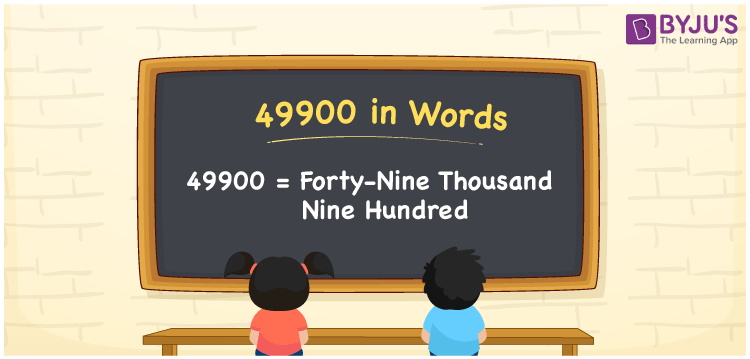# 49900 in Words

The number 49900 is represented by the word forty-nine thousand nine hundred. The number 49900 can be easily converted into words using the place value of a number. As a result, you must use a place value table to produce the number names. For example, You can write “I paid Rs. Forty-nine thousand nine hundred for purchasing a computer”, if you paid Rs. 49900 for purchasing a computer. You will learn how to spell 49900 in English as well as how to express the number in words in this article.

 49900 in Words: Forty-nine Thousand Nine Hundred. Forty-nine Thousand Nine Hundred in Numerical Form: 49900.

## 49900 in English Words## How to Write 49900 in Words?

The place value for the number 49900 is shown in the table below.

 Ten-thousands Thousands Hundreds Tens Ones 4 9 9 0 0

The expanded form of the number 49900 is as follows:

= 4 × Ten thousand + 9 × Thousand + 9 × Hundred + 0 × Ten + 0 × One

= 4 × 10000 + 9 × 1000 + 9 × 100

= 40000 + 9000 + 900

= 49900

= Forty-nine thousand nine hundred

Hence, 49900 in words is forty-nine thousand nine hundred.

49900 in words – Forty-nine thousand nine hundred

Is 49900 an odd number? – No

Is 49900 an even number? – Yes

Is 49900 a perfect square number? – No

Is 49900 a perfect cube number? – No

Is 49900 a prime number? – No

Is 49900 a composite number? – Yes

## Frequently Asked Questions on 49900 in Words

Q1

### How to write 49900 in words?

49900 in words is forty-nine thousand nine hundred.

Q2

### Simplify 49000 + 900, and express in words.

Simplifying 49000 + 900, we get 49900. Hence, 49900 in words is forty-nine thousand nine hundred.

Q3

### Convert forty-nine thousand nine hundred into numbers.

Forty-nine thousand nine hundred in numbers is 49900